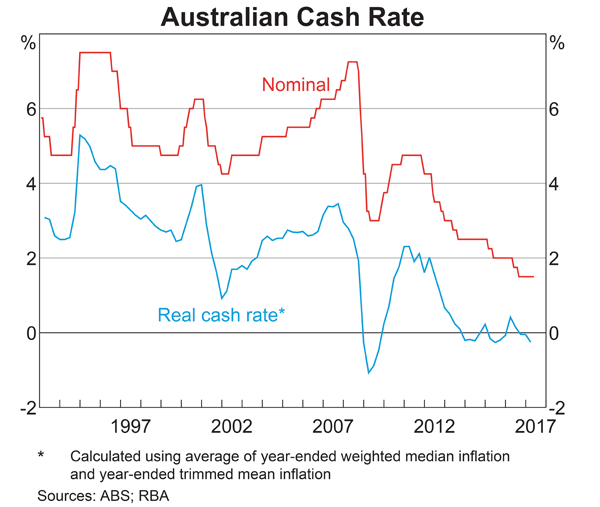Fight Finance

CoursesTagsRandomAllRecentScores

Economic statistics released this morning were a surprise: they show a strong chance of consumer price inflation (CPI) reaching 5% pa over the next 2 years.

This is much higher than the previous forecast of 3% pa.

A vanilla fixed-coupon 2-year risk-free government bond was issued at par this morning, just before the economic news was released.

What is the expected change in bond price after the economic news this morning, and in the next 2 years? Assume that:

• Inflation remains at 5% over the next 2 years.
• Investors demand a constant real bond yield.
• The bond price falls by the (after-tax) value of the coupon the night before the ex-coupon date, as in real life.

One method for calculating a firm's free cash flow (FFCF, or CFFA) is to ignore interest expense. That is, pretend that interest expense $(IntExp)$ is zero:

\begin{aligned} FFCF &= (Rev - COGS - Depr - FC - IntExp)(1-t_c) + Depr - CapEx -\Delta NWC + IntExp \\ &= (Rev - COGS - Depr - FC - 0)(1-t_c) + Depr - CapEx -\Delta NWC - 0\\ \end{aligned}
Does this annual FFCF with zero interest expense or the annual interest tax shield?

An expansion option is best modeled as a or option?

An investor bought a 10 year 2.5% pa fixed coupon government bond priced at par. The face value is $100. Coupons are paid semi-annually and the next one is in 6 months. Six months later, just after the coupon at that time was paid, yields suddenly and unexpectedly fell to 2% pa. Note that all yields above are given as APR's compounding semi-annually. What was the bond investors' historical total return over that first 6 month period, given as an effective semi-annual rate? A stock has an arithmetic average continuously compounded return (AALGDR) of 10% pa, a standard deviation of continuously compounded returns (SDLGDR) of 80% pa and current stock price of$1. Assume that stock prices are log-normally distributed.

In one year, what do you expect the mean and median prices to be? The answer options are given in the same order.

Five years ago ($t=-5$ years) you entered into an interest-only home loan with a principal of \$500,000, an interest rate of 4.5% pa compounding monthly with a term of 25 years.

Then interest rates suddenly fall to 3% pa ($t=0$), but you continue to pay the same monthly home loan payments as you did before. Will your home loan be paid off by the end of its remaining term? If so, in how many years from now? Measure the time taken to pay off the home loan from the current time which is 5 years after the home loan was first entered into.

Assume that the lower interest rate was given to you immediately after the loan repayment at the end of year 5, which was the 60th payment since the loan was granted. Also assume that rates were and are expected to remain constant.

Which of the following statements about an option (either a call or put) and its underlying stock is NOT correct?

The Australian central bank implements monetary policy by directly controlling which interest rate?

Below is the Australian central bank’s cash rate.From 2011 to 2017 the Australian central bank has implemented:

Question 904  option, Black-Scholes-Merton option pricing, option on future on stock index

A six month European-style call option on six month S&P500 index futures has a strike price of 2800 points.

The six month futures price on the S&P500 index is currently at 2740.805274 points. The futures underlie the call option.

The S&P500 stock index currently trades at 2700 points. The stock index underlies the futures. The stock index's standard deviation of continuously compounded returns is 25% pa.

The risk-free interest rate is 5% pa continuously compounded.

Use the Black-Scholes-Merton formula to calculate the option price. The call option price now is: